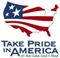Duration Plots

# Flow-Duration Plot description

Flow-duration plots depict specific flow-statistic values selected from flow-duration curves. The flow-duration curve is a cumulative frequency curve that shows the percent of values that specified discharges were equaled or less than (percentile), or the percent of values that specified discharges were equaled or exceeded (percent exceedance). Percent exceedance is 100 minus the percentile. Example: the 75th percent exceedance (the value that 75 percent of the flows are equal to or greater than) is the 25th percentile (the value that 25 percent of the flows are equal to or less than). While the flow-duration curve does not show the chronological sequence of flows, it is an important means of representing flow characteristics of a stream throughout the range of flow on a single curve.

The flow-duration plots presented here represent the flow-duration curves of daily streamflow data grouped by months. All the daily flow values for each month are arranged according to magnitude and the percentile for each daily flow value is computed. The value for a specific percentile is determined by interpolation between the computed values. From the data, the 5th, 10th, 25th, 50th, 75th, 90th and 95th percentiles are determined and plotted. Colors are used to separate above normal (blue), normal (green), and below normal (brown) conditions. The maximum and minimum daily values for each month are selected from the data set. The instantaneous minimum is determined from historic flow records.U.S. Department of the Interior | U.S. Geological Survey
URL: http://va.water.usgs.gov/duration_plots/fdp_description.htm
Page Contact Information: Virginia WSC Webmaster